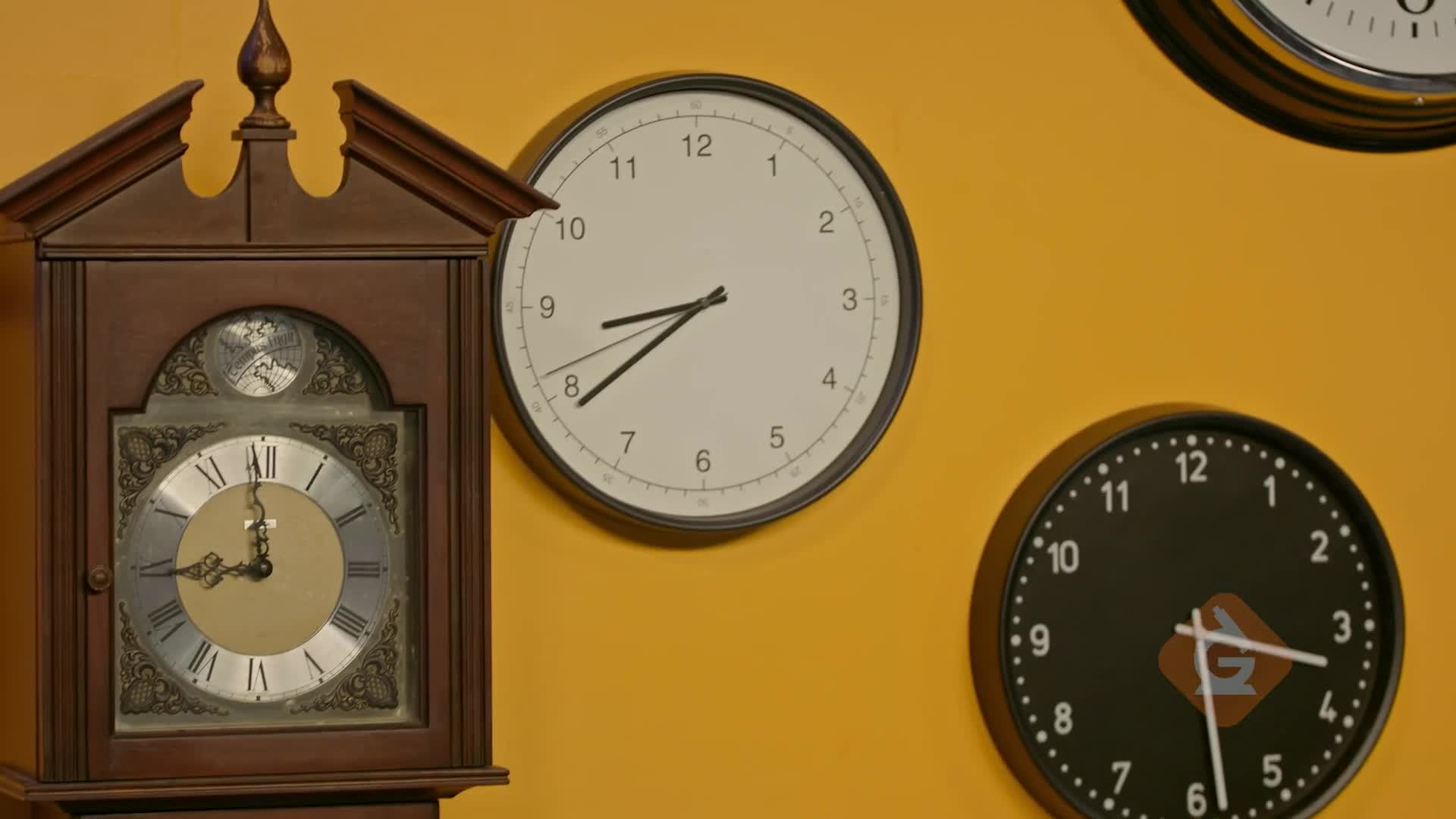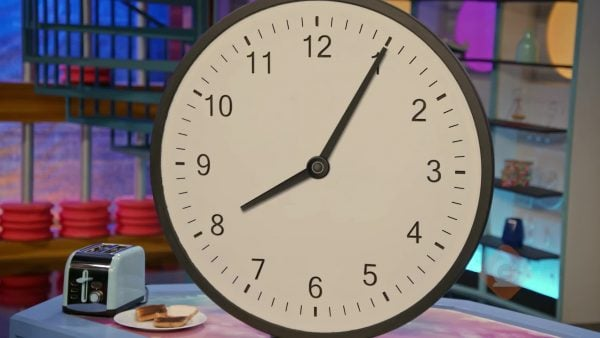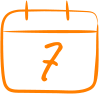Tell Time (Nearest Minute) Math Video for Kids - 3rd, 4th, & 5th Grades
1%
It was processed successfully!WHAT IS TELLING TIME TO THE NEAREST MINUTE?

The minute hand tells you how many minutes past the hour. The lines on a clock show 5 minutes at a time. You can skip count by fives to find how many minutes.

To better understand telling time to the nearest minute…

WHAT IS TELLING TIME TO THE NEAREST MINUTE?. The minute hand tells you how many minutes past the hour. The lines on a clock show 5 minutes at a time. You can skip count by fives to find how many minutes. To better understand telling time to the nearest minute…

## LET’S BREAK IT DOWN!

### Counting NumbersKnowing how to tell time to the nearest 5 minutes can keep you from burning your snack. Suppose you are baking some chicken fingers. The time is 3:00. The chicken fingers will be done at 3:20. What number on the clock will the minute hand point to at 3:20? Each number on the clock represents 5 minutes. So, you can start at 12 and count by 5s until you get to 20. 1 is 5 minutes, 2 is 10 minutes, 3 is 15 minutes, and 4 is 20 minutes. This means the minute hand will be pointing to the 4 at 3:20. That’s when you can take the chicken fingers out of the oven. Try this one yourself. Suppose your pizza is in the oven and will be done at 5:40. What number on the clock will the minute hand point to when you can take the pizza out of the oven?

Counting Numbers Knowing how to tell time to the nearest 5 minutes can keep you from burning your snack. Suppose you are baking some chicken fingers. The time is 3:00. The chicken fingers will be done at 3:20. What number on the clock will the minute hand point to at 3:20? Each number on the clock represents 5 minutes. So, you can start at 12 and count by 5s until you get to 20. 1 is 5 minutes, 2 is 10 minutes, 3 is 15 minutes, and 4 is 20 minutes. This means the minute hand will be pointing to the 4 at 3:20. That’s when you can take the chicken fingers out of the oven. Try this one yourself. Suppose your pizza is in the oven and will be done at 5:40. What number on the clock will the minute hand point to when you can take the pizza out of the oven?

### Counting TicksKnowing how to tell time to the nearest minute can help make sure you do not miss your bus. Suppose a clock shows that the time your bus picks you up is when the hour hand is between 7 and 8 and the minute hand is 2 dashes past 7. You can figure out what time the bus picks you up by first naming the hour. The hour hand is between 7 and 8, so the hour is 7 because you always choose the number that comes first. Each number on the clock represents 5 minutes, so you can count by 5s to 7: 1 is 5 minutes, 2, is 10 minutes, 3 is 15 minutes, 4 is 20 minutes, 5 is 25 minutes, 6 is 30 minutes, and 7 is 35 minutes. Each dash represents 1 minute. So, start at 35 and count on by 1s to get the minutes: 35, 36, 37. The bus picks you up at 7:37. Try this one yourself. Suppose a clock shows that the time your bus drops you off after school is when the hour hand is between 3 and 4 and the minute hand is 3 dashes past 2. What time does the bus drop you off?

Counting Ticks Knowing how to tell time to the nearest minute can help make sure you do not miss your bus. Suppose a clock shows that the time your bus picks you up is when the hour hand is between 7 and 8 and the minute hand is 2 dashes past 7. You can figure out what time the bus picks you up by first naming the hour. The hour hand is between 7 and 8, so the hour is 7 because you always choose the number that comes first. Each number on the clock represents 5 minutes, so you can count by 5s to 7: 1 is 5 minutes, 2, is 10 minutes, 3 is 15 minutes, 4 is 20 minutes, 5 is 25 minutes, 6 is 30 minutes, and 7 is 35 minutes. Each dash represents 1 minute. So, start at 35 and count on by 1s to get the minutes: 35, 36, 37. The bus picks you up at 7:37. Try this one yourself. Suppose a clock shows that the time your bus drops you off after school is when the hour hand is between 3 and 4 and the minute hand is 3 dashes past 2. What time does the bus drop you off?

### All Types of ClocksOnce you know how to read one analog clock, you can read any clock. Some clocks have different symbols for the numbers. There is even a clock that uses dominos for the numbers. Telling the time works the same no matter what the symbols are. There are 12 symbols, each 5 minutes apart. Start by finding where the 1 should be on the clock and think of that symbol as 1, the second symbol as 2, and continue around the clock until you get to 12 so you can use the numbers you are used to. This can work even if the clock has no numbers because you can place the numbers where they should be. Suppose the hour hand is between the symbols V and VI, which are Roman numerals for 5 and 6. And suppose the minute hand is pointing to X, which is the Roman numeral for 10. What time is on the clock? The hour is 5 because the hour hand is between 5 and 6 and 5 is the number that comes first. If you count by 5s from the first symbol to 10, that is 50. So, the time is 5:50. Try this one yourself. Suppose a clock has no numbers on it. The minute hand points straight up and the hour hand points to the right. What time is on the clock? You can draw a picture to help you.

All Types of Clocks Once you know how to read one analog clock, you can read any clock. Some clocks have different symbols for the numbers. There is even a clock that uses dominos for the numbers. Telling the time works the same no matter what the symbols are. There are 12 symbols, each 5 minutes apart. Start by finding where the 1 should be on the clock and think of that symbol as 1, the second symbol as 2, and continue around the clock until you get to 12 so you can use the numbers you are used to. This can work even if the clock has no numbers because you can place the numbers where they should be. Suppose the hour hand is between the symbols V and VI, which are Roman numerals for 5 and 6. And suppose the minute hand is pointing to X, which is the Roman numeral for 10. What time is on the clock? The hour is 5 because the hour hand is between 5 and 6 and 5 is the number that comes first. If you count by 5s from the first symbol to 10, that is 50. So, the time is 5:50. Try this one yourself. Suppose a clock has no numbers on it. The minute hand points straight up and the hour hand points to the right. What time is on the clock? You can draw a picture to help you.

## TELLING TIME (NEAREST MINUTE) VOCABULARY

Clock
A tool used to measure time by using hands on a round dial or by showing digits.
Roman numerals
A number system that started in ancient Rome.
Grandfather clock
A clock in a tall case that runs using a pendulum and weights.
Analog clock
A tool for reading time with a shorter hand that shows the hour and a longer hand that shows the minutes.
Digital clock
A clock that shows the time by using digits.
Digits
Any numeral from 0 to 9: 0, 1, 2, 3, 4, 5, 6, 7, 8, 9.
The hour hand that shows the hours on a clock.
A unit of time that is 60 minutes.
The minute hand that shows the minutes on a clock.
A unit of time, 60 minutes is 1 hour.
Means ‘of the clock’; used to name a time that is exactly on the hour.
Any time before noon.
Any time after noon.
12:00 in the afternoon, midday.

## TELLING TIME (NEAREST MINUTE) DISCUSSION QUESTIONS

### How many minutes are in 1 hour? Does each number on the clock represent 1 minute?

There are 60 minutes in 1 hour. No, each number on the clock represents 5 minutes.

### Show the time 1:50 on the analog demonstration clock. Is the time 1:10? Explain.

No, the minute hand is pointing to the 10. You count by 5s to the number 10 to find the minutes: 5, 10, 15, 20, 25, 30, 35, 40, 45, 50. The time is 1:50.

### Explain where you would draw the hands to show 7:17.

The hour is 7, so the hour hand goes between 7 and 8. For the minute hand, count to the nearest 5: 1 is 5 minutes, 2 is 10 minutes, and 3 is 15 minutes. 17 is 2 dashes past 15, so count 2 dashes past the number 3 and draw the minute hand.

### Would you get the same answer if you started the minute hand at 12 and just counted 17 dashes? Explain.

Yes, counting 17 dashes ends at the same place on the clock. Each number represents 5, so counting by 5s to the nearest 5 is quicker and easier than counting by 1s.

### Show 12:38 on the analog demonstration clock. Explain how to find the time on the clock.

The hour hand is between 12 and 1, so the hour is 12. The minute hand is pointing to 3 dashes past 7. Count by 5s to 7: 5, 10, 15, 20, 25, 30, 35. Count on 3: 35, 36, 37, 38. The time is 12:38.
X

## Success

We’ve sent you an email with instructions how to reset your password.
Ok

## Choose Your Free Trial Period### 7 DaysContinue to Lessons### 90 DaysGet 90 days free by inviting other teachers to try it too.

Share with Teachers

## Get 90 Days Free

### By inviting 4 other teachers to try it too.

4 required

Skip, I will use a 7 days free trial

## Thank You!

Enjoy your free 90 days trial